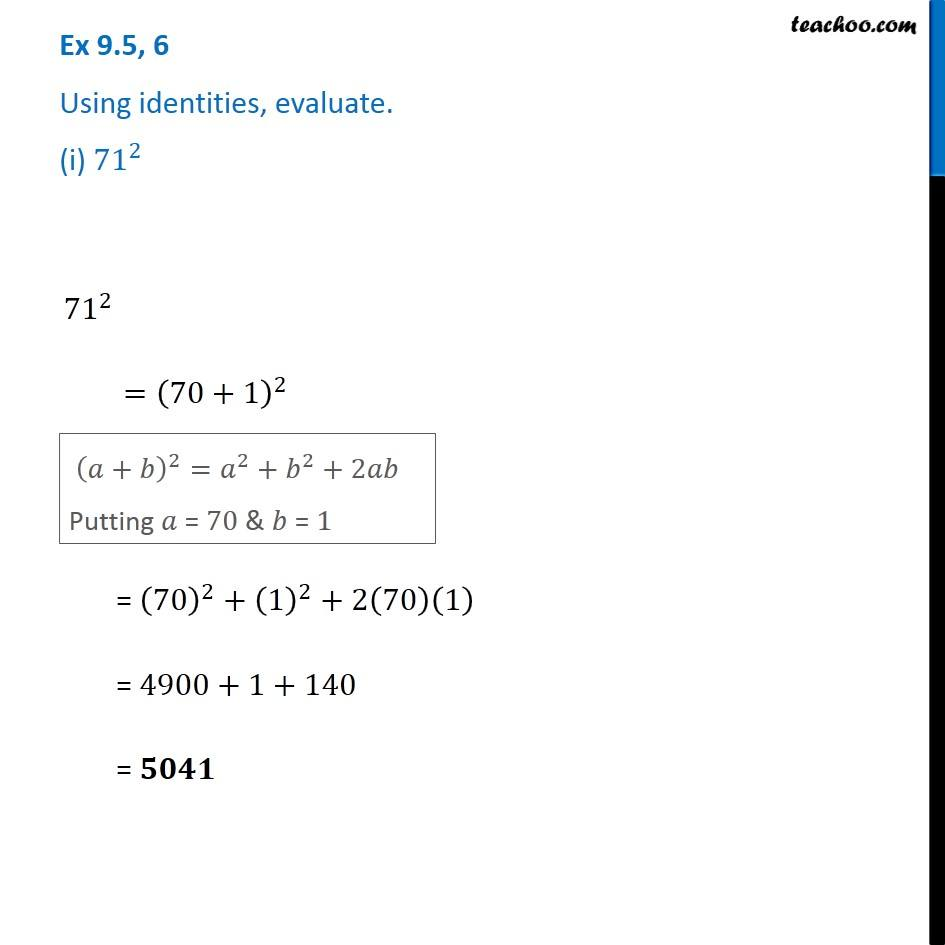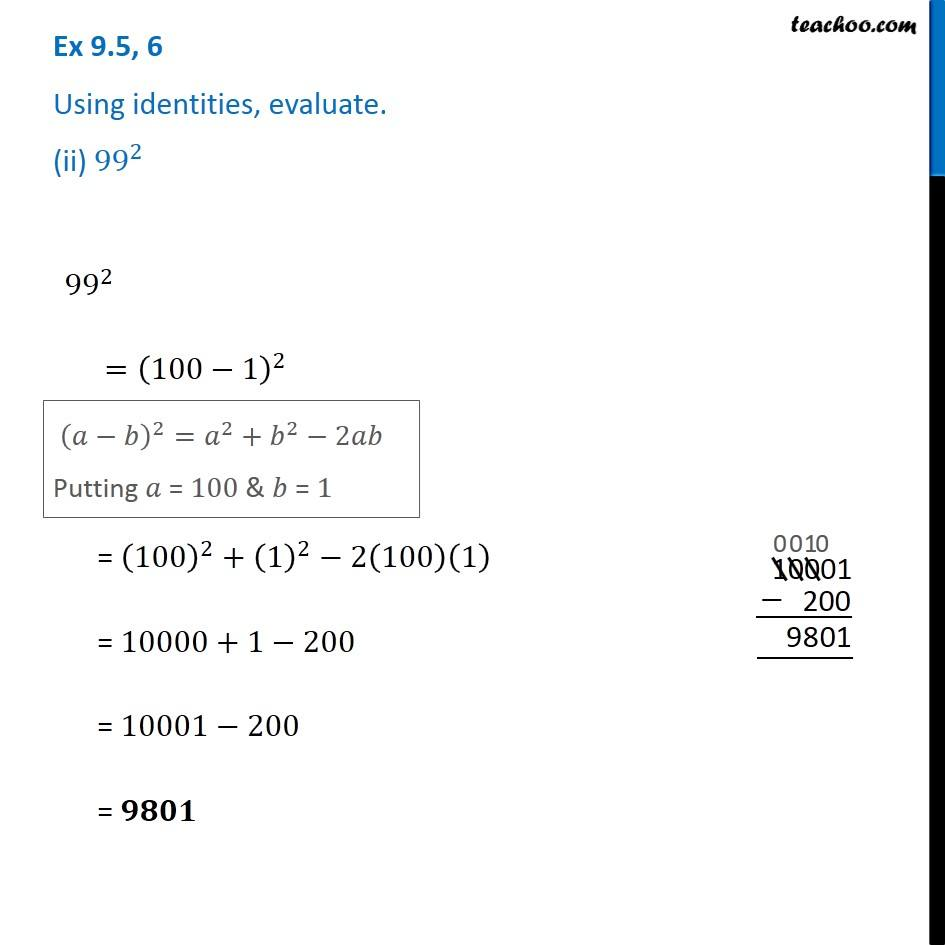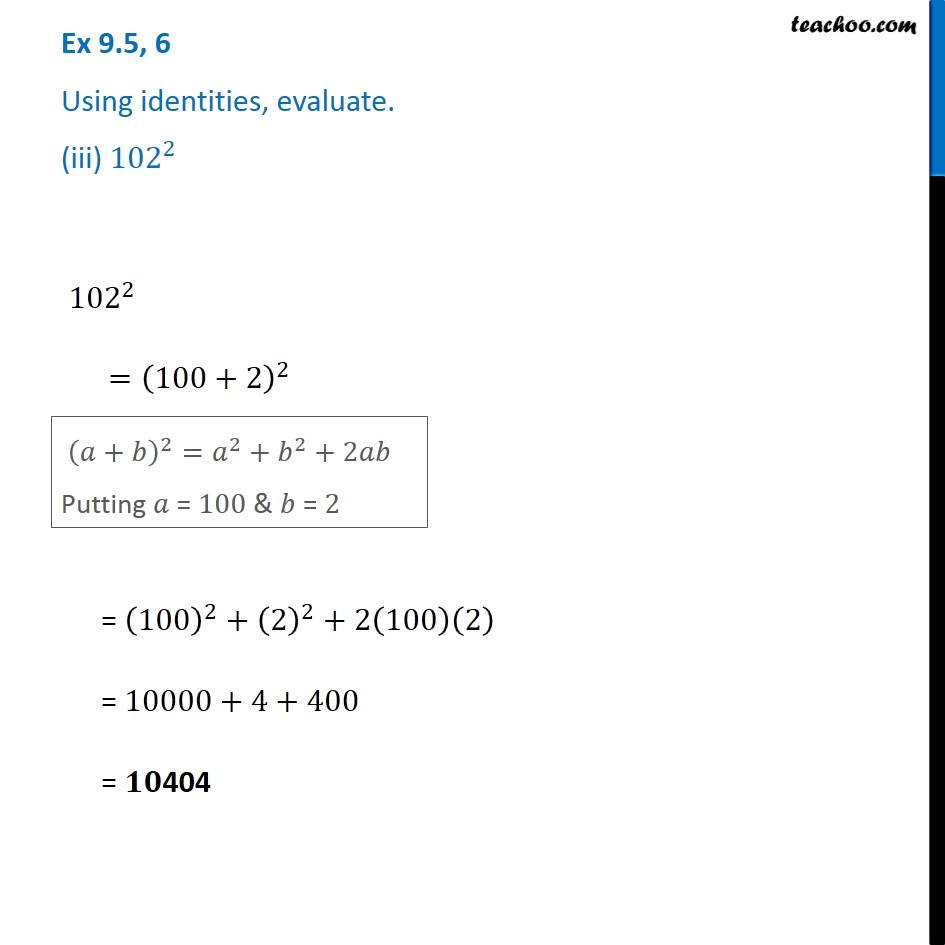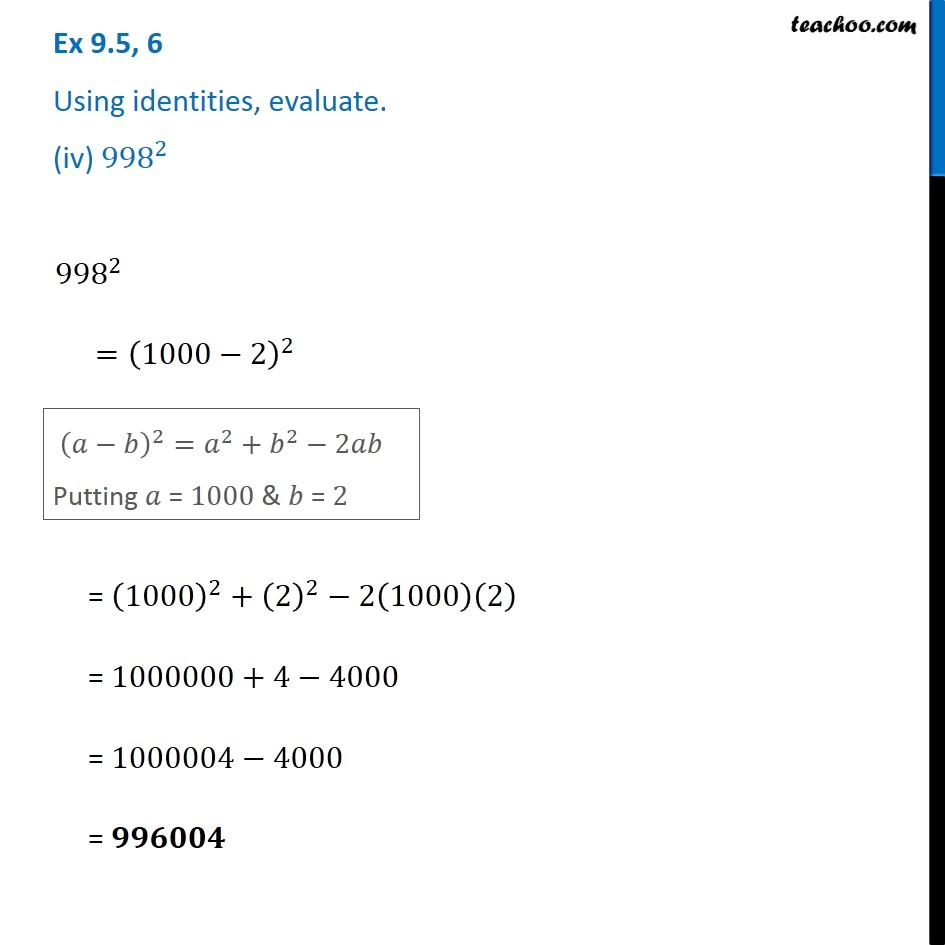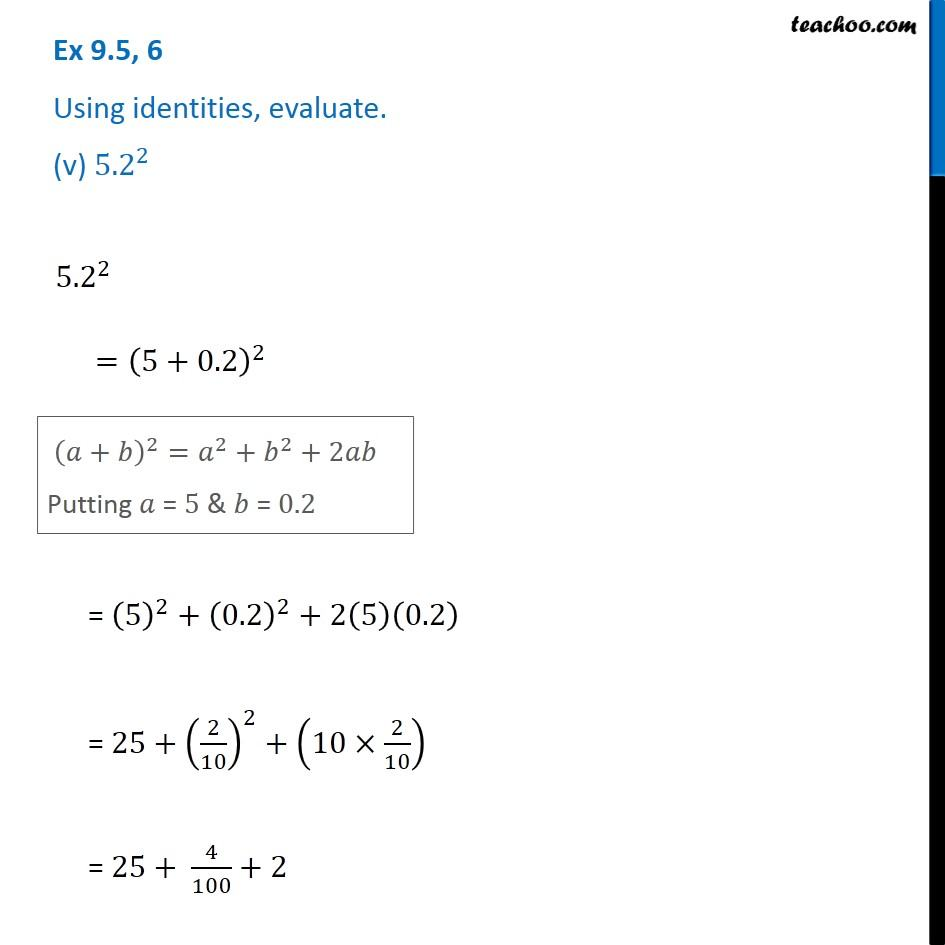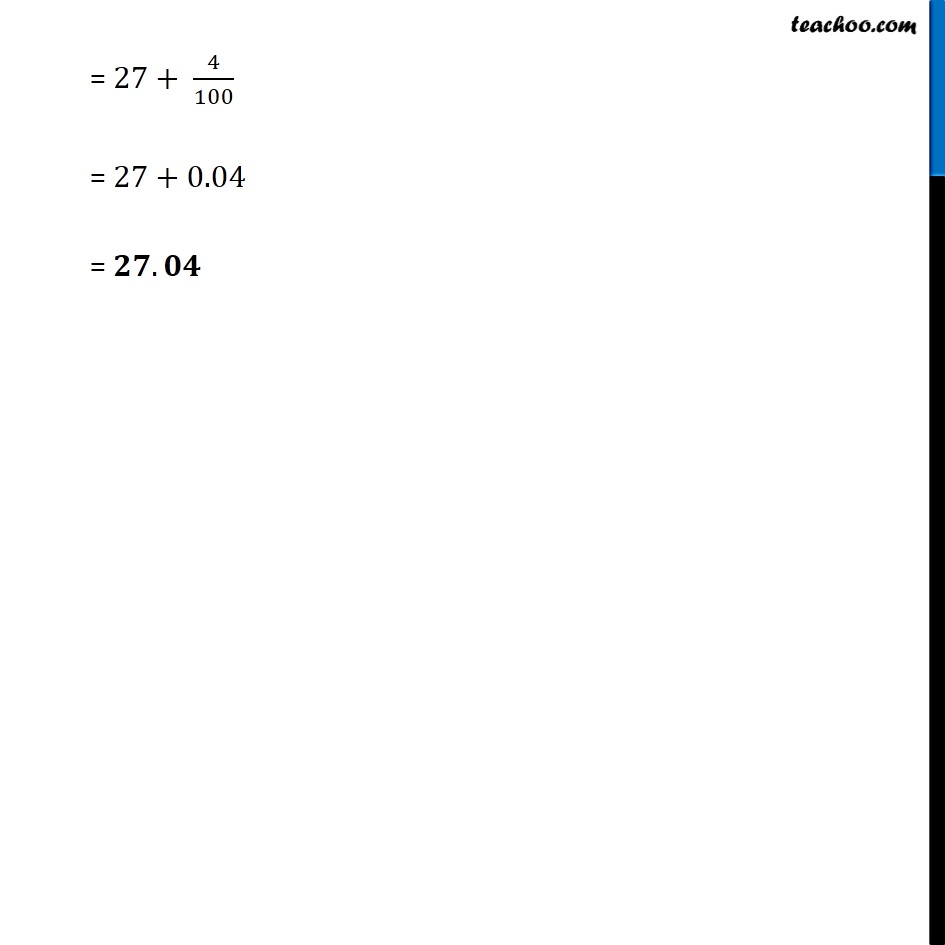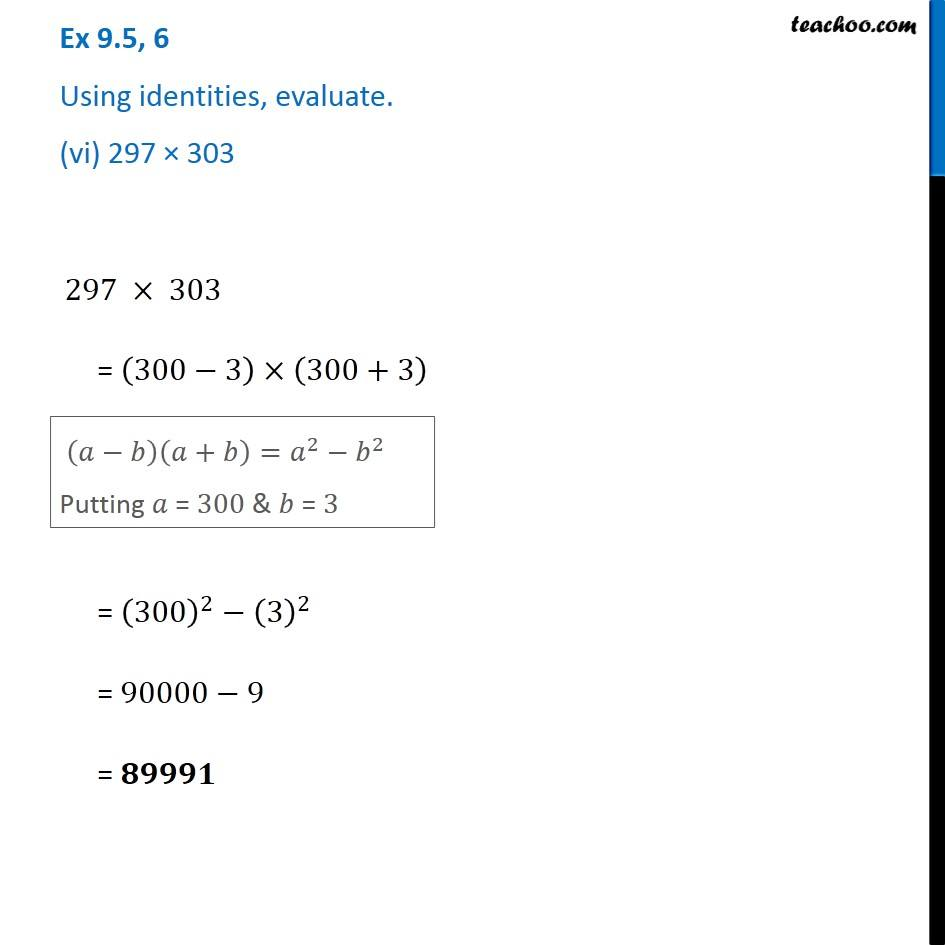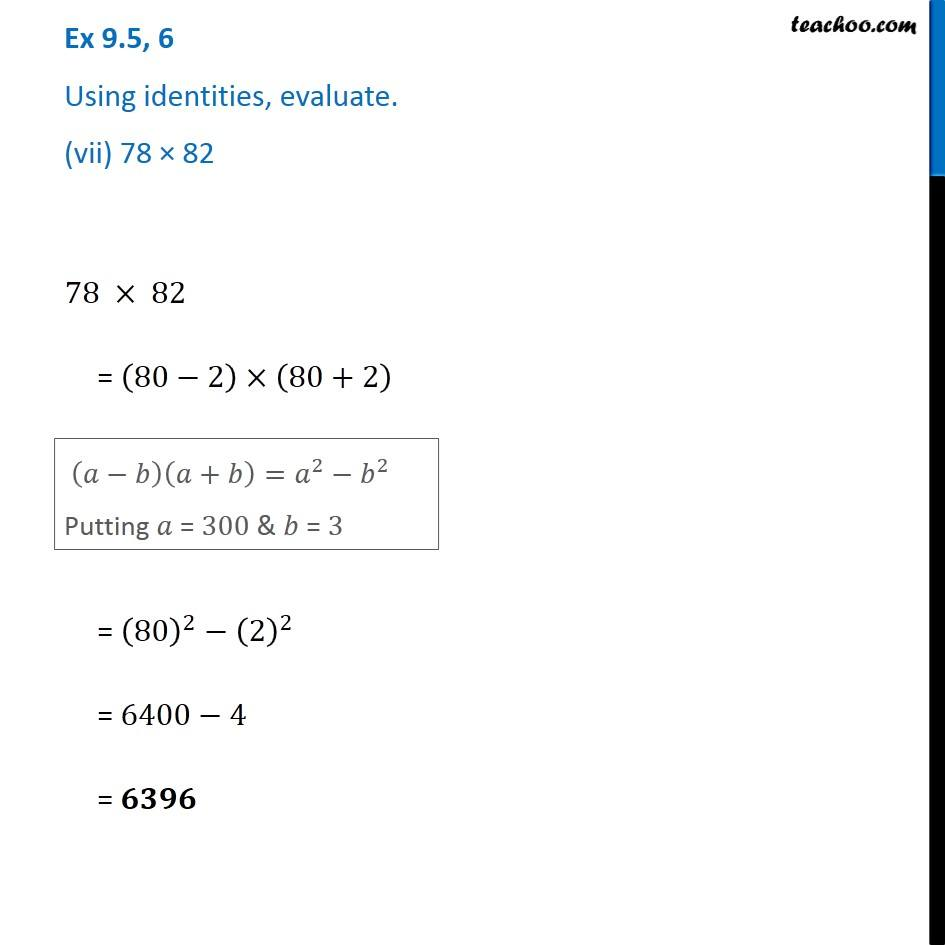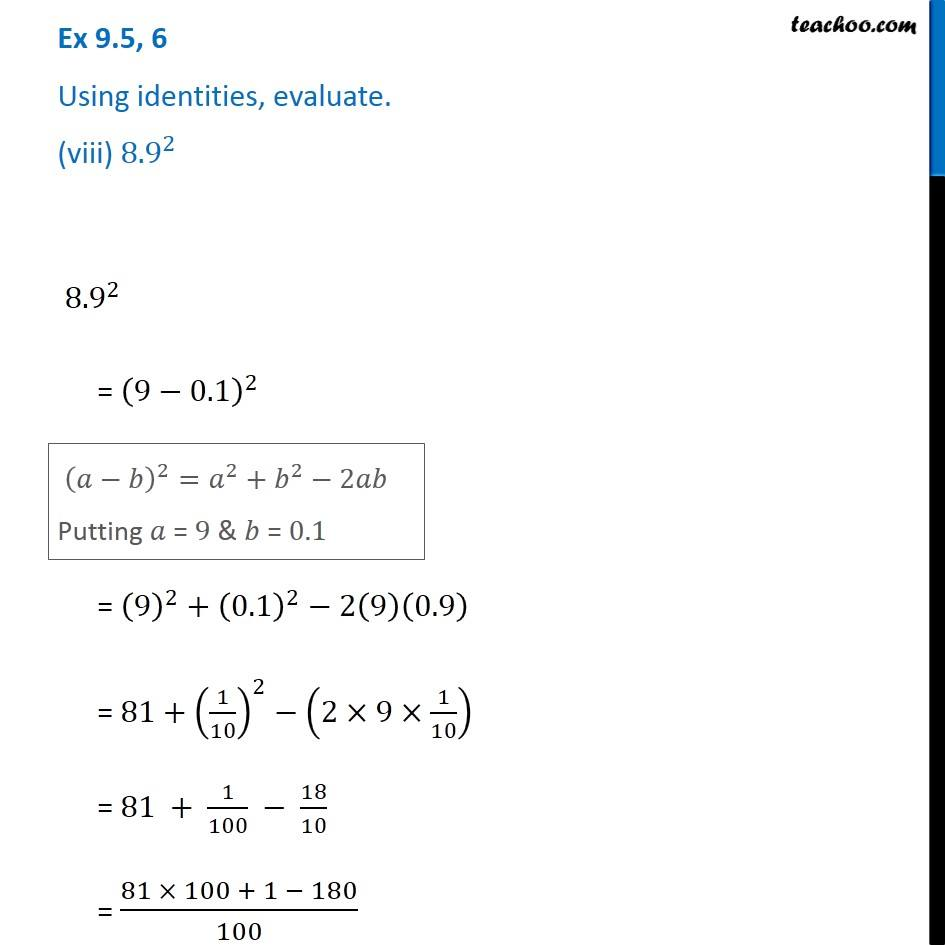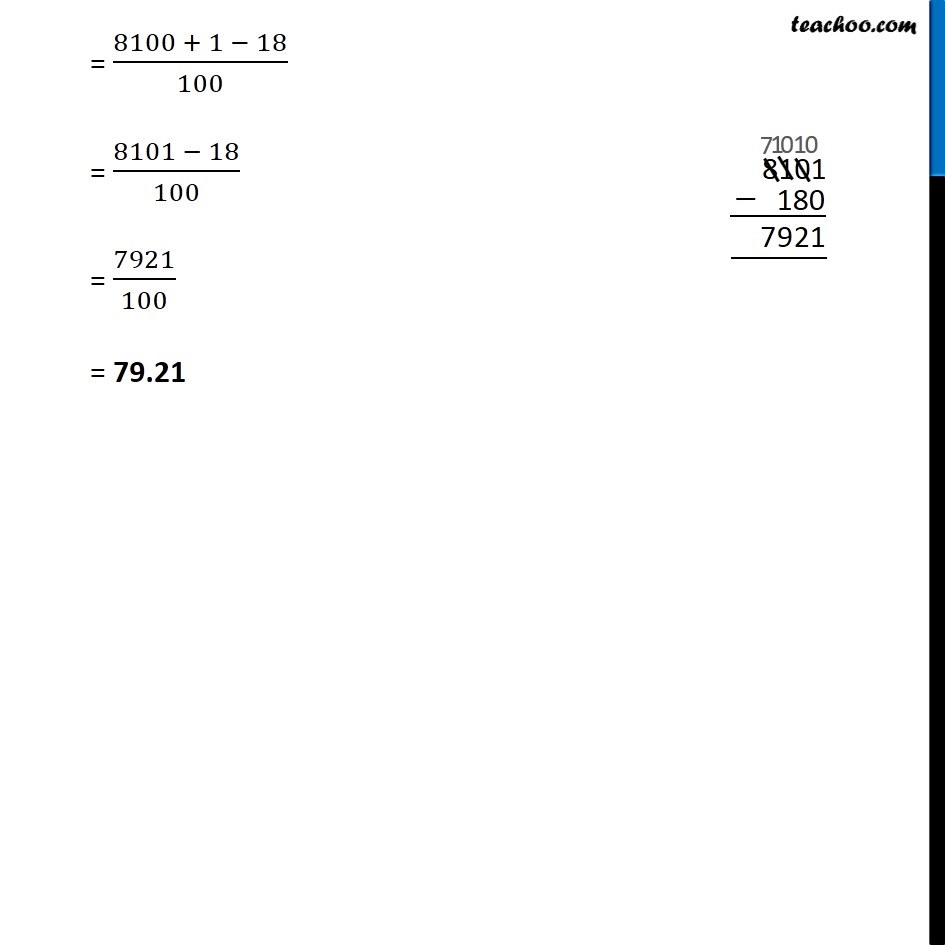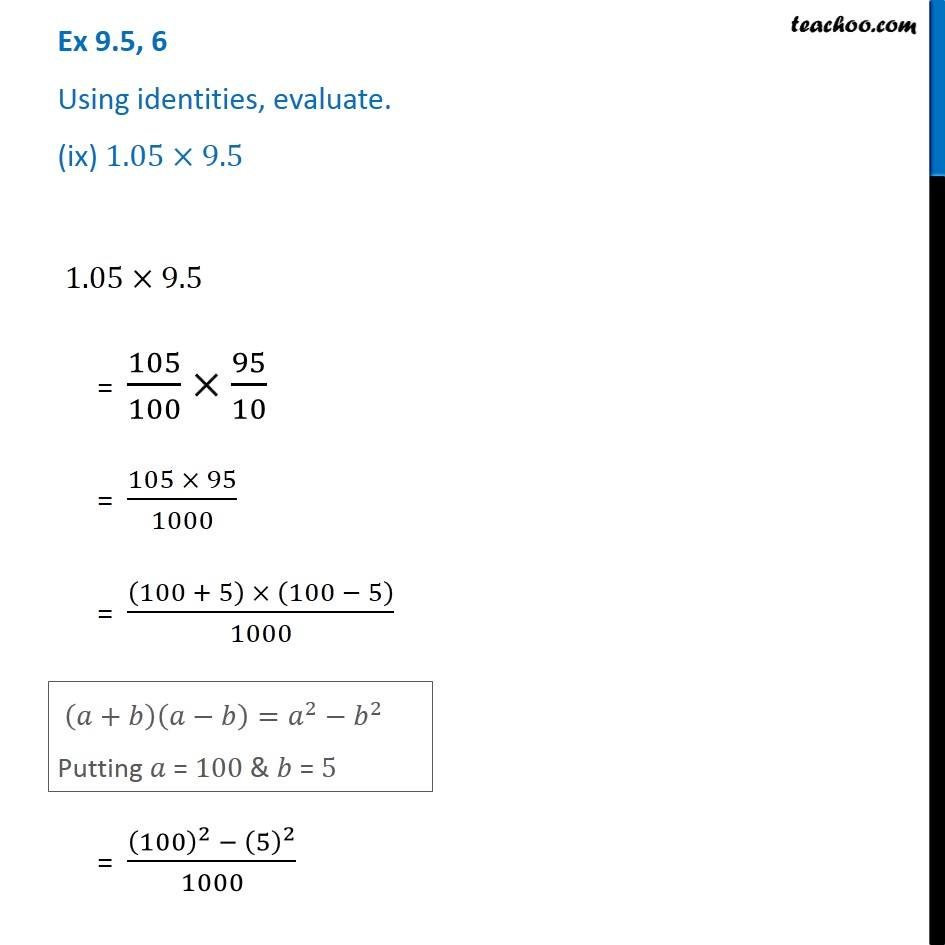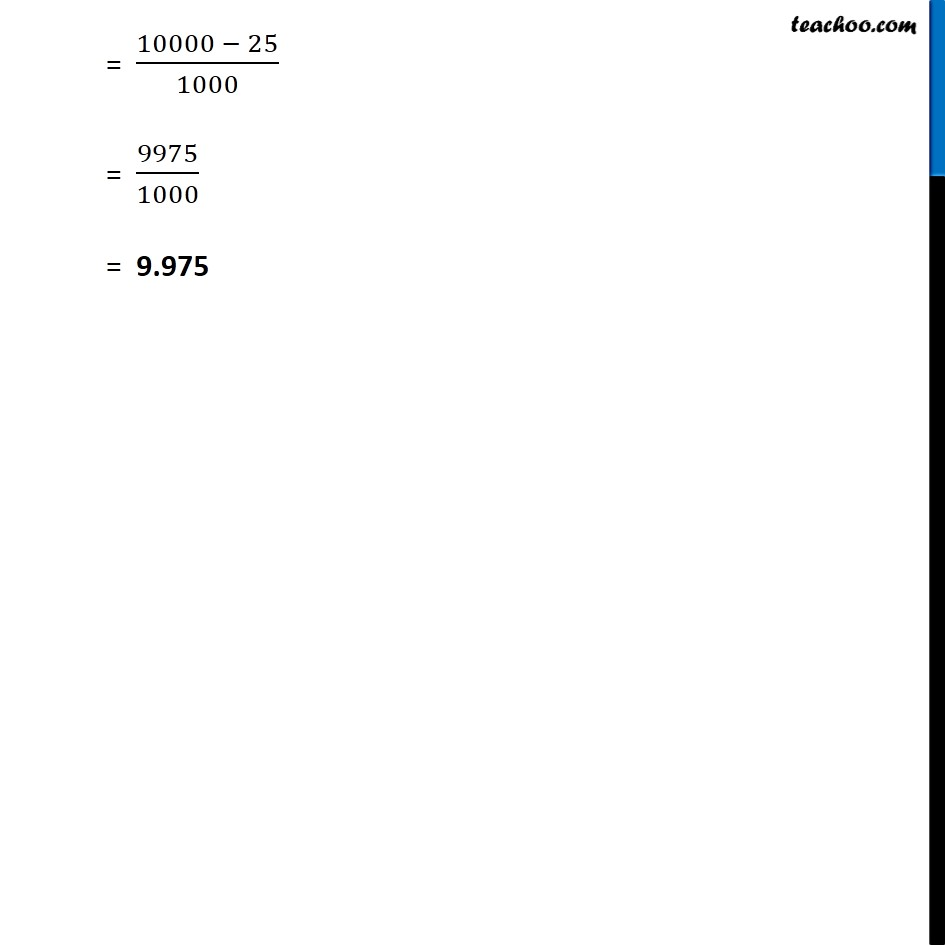1. Chapter 9 Class 8 Algebraic Expressions and Identities
2. Serial order wise
3. Ex 9.5

Transcript

Ex 9.5, 6 Using identities, evaluate. (i) 〖71〗^2 〖71〗^2 =(70+1)^2 (𝑎+𝑏)^2=𝑎^2+𝑏^2+2𝑎𝑏 Putting 𝑎 = 70 & 𝑏 = 1 = (70)^2+(1)^2+2(70)(1) = 4900+1+140 = 𝟓𝟎𝟒𝟏 Ex 9.5, 6 Using identities, evaluate. (ii) 〖99〗^2 〖99〗^2 =(100−1)^2 (𝑎−𝑏)^2=𝑎^2+𝑏^2−2𝑎𝑏 Putting 𝑎 = 100 & 𝑏 = 1 = (100)^2+(1)^2−2(100)(1) = 10000+1−200 = 10001−200 = 𝟗𝟖𝟎𝟏 Ex 9.5, 6 Using identities, evaluate. (iii) 〖102〗^2 〖102〗^2 =(100+2)^2 (𝑎+𝑏)^2=𝑎^2+𝑏^2+2𝑎𝑏 Putting 𝑎 = 100 & 𝑏 = 2 = (100)^2+(2)^2+2(100)(2) = 10000+4+400 = 𝟏𝟎404 Ex 9.5, 6 Using identities, evaluate. (iv) 〖998〗^2 〖998〗^2 =(1000−2)^2 (𝑎−𝑏)^2=𝑎^2+𝑏^2−2𝑎𝑏 Putting 𝑎 = 1000 & 𝑏 = 2 = (1000)^2+(2)^2−2(1000)(2) = 1000000+4−4000 = 1000004−4000 = 𝟗𝟗𝟔𝟎𝟎𝟒 Ex 9.5, 6 Using identities, evaluate. (v) 〖5.2〗^2 〖5.2〗^2 =(5+0.2)^2 (𝑎+𝑏)^2=𝑎^2+𝑏^2+2𝑎𝑏 Putting 𝑎 = 5 & 𝑏 = 0.2 (𝑎+𝑏)^2=𝑎^2+𝑏^2+2𝑎𝑏 Putting 𝑎 = 5 & 𝑏 = 0.2 = 27+ 4/100 = 27+0.04 = 𝟐𝟕.𝟎𝟒 Ex 9.5, 6 Using identities, evaluate. (vi) 297 × 303 297 × 303 = (300−3)×(300+3) (𝑎−𝑏)(𝑎+𝑏)=𝑎^2−𝑏^2 Putting 𝑎 = 300 & 𝑏 = 3 = (300)^2−(3)^2 = 90000−9 = 𝟖𝟗𝟗𝟗𝟏 Ex 9.5, 6 Using identities, evaluate. (vii) 78 × 82 78 × 82 = (80−2)×(80+2) (𝑎−𝑏)(𝑎+𝑏)=𝑎^2−𝑏^2 Putting 𝑎 = 300 & 𝑏 = 3 = (80)^2−(2)^2 = 6400−4 = 𝟔𝟑𝟗𝟔 Ex 9.5, 6 Using identities, evaluate. (viii) 〖8.9〗^2 〖8.9〗^2 = (9−0.1)^2 (𝑎−𝑏)^2=𝑎^2+𝑏^2−2𝑎𝑏 Putting 𝑎 = 9 & 𝑏 = 0.1 = (9)^2+(0.1)^2−2(9)(0.9) = 81+(1/10)^2−(2×9×1/10) = 81 + 1/100 − 18/10 = (81 × 100 + 1 − 180)/100 = (8100 + 1 − 18)/100 = (8101 − 18)/100 = 7921/100 = 79.21 Ex 9.5, 6 Using identities, evaluate. (ix) 1.05×9.5 1.05×9.5 = 105/100×95/10 = (105 × 95)/1000 = ((100 + 5) × (100 − 5))/1000 (𝑎+𝑏)(𝑎−𝑏)=𝑎^2−𝑏^2 Putting 𝑎 = 100 & 𝑏 = 5 = ((100)^2 − (5)^2)/1000 = (10000 − 25)/1000 = 9975/1000 = 9.975

Ex 9.5